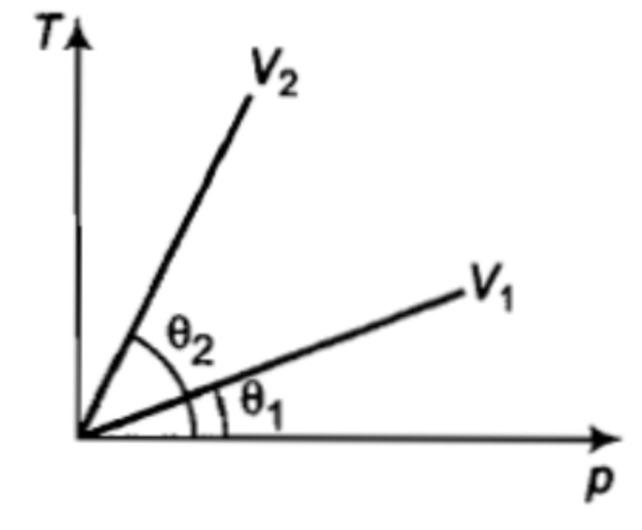Gases exert pressure on the walls of containing vessel because  the gas molecules:

(1) Possess momentum

(2) collide with each other

(3) have finite volume

(4) obey gas laws

Concept Questions :-

Kinetic energy of gas
Maxwell distribution curve
High Yielding Test Series + Question Bank - NEET 2020

Difficulty Level:

The equation of state for 5 g of oxygen at a pressure P and temperature T, when occupying a volume V, will be: (where R is the constant)

(1) PV = 5RT

(2) PV = $\left(\frac{5}{2}\right)RT$

(3) PV = $\left(\frac{5}{16}\right)RT$

(4) PV = $\left(\frac{5}{32}\right)RT$

Concept Questions :-

Ideal gas
High Yielding Test Series + Question Bank - NEET 2020

Difficulty Level:

From the P - T graph, what conclusion can be drawn?(1) ${V}_{2}={V}_{1}$

(2) ${V}_{2}<{V}_{1}$

(3) ${V}_{2}>{V}_{1}$

(4) Nothing can be predicted

Concept Questions :-

Ideal gas
High Yielding Test Series + Question Bank - NEET 2020

Difficulty Level:

The root mean square speed of the molecules of an enclosed gas is V.  What will be the root mean square speed if the pressure is doubled, the temperature remaining the same?

(1) $\frac{v}{2}$      (2) v          (3) 2        (4) 4 v

Concept Questions :-

Types of velocity
High Yielding Test Series + Question Bank - NEET 2020

Difficulty Level:

If the degree of freedom of gas are f, then the ratio of two specific heats ${C}_{P}/{C}_{V}$ is given by:

[MP PET 1995; BHU 1997; MP PMT 2001, 04]

(1) $\frac{2}{f}+1$                   (2) $1-\frac{2}{f}$

(3) $1+\frac{1}{f}$                   (4) $1-\frac{1}{f}$

Concept Questions :-

Specific heat
High Yielding Test Series + Question Bank - NEET 2020

Difficulty Level:

The equation $\left(p+\frac{a}{{v}^{2}}\right)\left(v-b\right)=RT$ is known as:

(1) Perfect gas equation

(2) Joule Thomson's equation

(3) Vander Waal's equation

(4) Maxwell's equation

Concept Questions :-

Ideal gas
High Yielding Test Series + Question Bank - NEET 2020

Difficulty Level:

The temperature of an ideal gas is increased from $27°$ to $927°C$. The r.m.s. speed of its molecules becomes-

(1) twice            (2) half            (3) four times          (4) one fourth

Concept Questions :-

Types of velocity
High Yielding Test Series + Question Bank - NEET 2020

Difficulty Level:

An ideal gas is filled in a vessel, then

(1) If it is placed inside a moving train, its temperature increases

(2) Its centre of mass moves randomly

(3) Its temperature remains constant in a moving car

(4) None of these

Concept Questions :-

Kinetic energy of gas
High Yielding Test Series + Question Bank - NEET 2020

Difficulty Level:

If the molecular weight of two gases are , then at a temperature the ratio of root mean square velocity  will be: [MP PMT 1996; AMU (Engg.) 2000; CPMT 2000; DPMT 2001]

(1) $\sqrt{\frac{{M}_{1}}{{M}_{2}}}$                          (2) $\sqrt{\frac{{M}_{2}}{{M}_{1}}}$

(3) $\sqrt{\frac{{M}_{1}+{M}_{2}}{{M}_{1}-{M}_{2}}}$                  (4) $\sqrt{\frac{{M}_{1}-{M}_{2}}{{M}_{1}+{M}_{2}}}$

Concept Questions :-

Types of velocity
High Yielding Test Series + Question Bank - NEET 2020

Difficulty Level:

An ideal gas is found to obey an additional law $V{P}^{2}$= constant. The gas is initially at temperature T and volume V. When it expands to a volume 2 V, the temperature becomes:

(1) T $\sqrt{2}$         (2) 2T         (3) 2T$\sqrt{2}$           (4) 4T

Concept Questions :-

Ideal gas